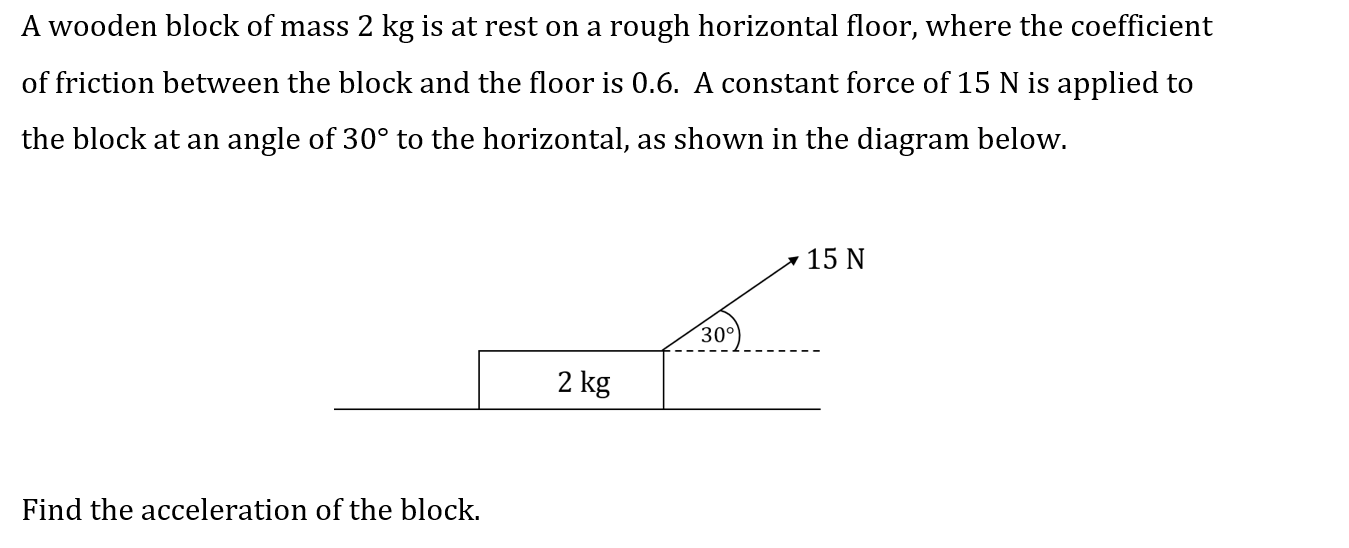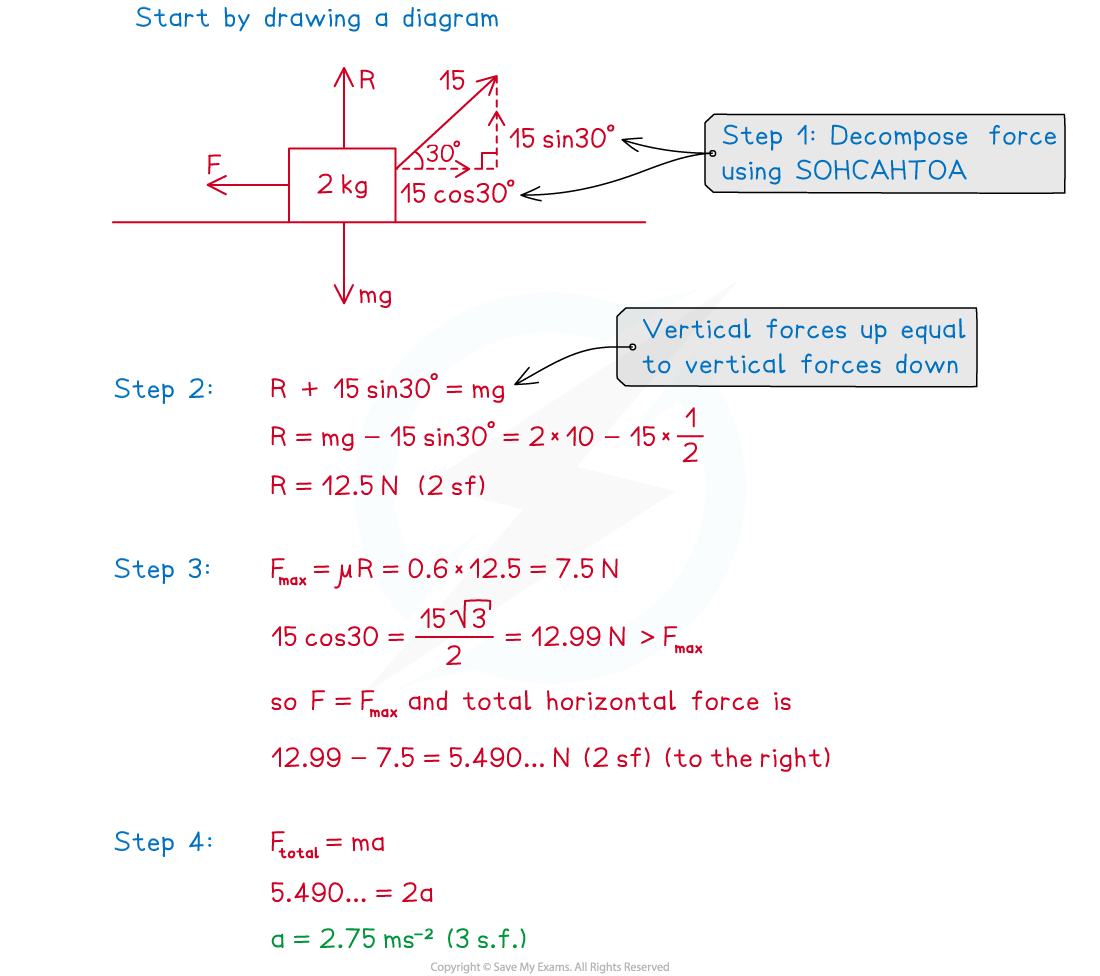# CIE A Level Maths: Mechanics复习笔记3.3.3 Coefficient of Friction - F = ma

### Coefficient of Friction - F = ma

#### How do we apply Newton’s Second Law (F = ma) in problems involving friction?

• The coefficient of friction combined with ma allows you to determine an object's motion where friction is involved in a problem
• For problems where the surface is horizontal:

Step 1. If necessary, resolve any angled forces into vertical and horizontal components

Step 2. Calculate the normal reaction force R

• Be careful – if there are vertical forces other than gravity these will affect the value of R
• with a horizontal surface R will always be directed vertically upwards
• the magnitude of will be such as to make the total make the total vertical force on the object zero

Step 3. Calculate FMAXμR and find the resultant (total force) of all the horizontal forces on the object

• Remember – if the resultant of the other horizontal forces is less than or equal to FMAX then friction will exactly balance those forces out and the object will remain stationary

Step 4. Use F = ma to determine the acceleration of the object

• For non-horizontal surfaces see the notes on inclined planes

#### Worked Example#### Exam Tip

• Always draw a force diagram and label it clearly.Look out for the words smooth and rough in mechanics problems involving an object moving (or potentially moving) along a surface:
• If the surface is described as smooth then you can ignore friction in the problem (ie μ= 0)
• If the surface is described as rough than you need to include the force of friction in solving the problem
• Be aware of whether the question is on a horizontal surface or an inclined plane.